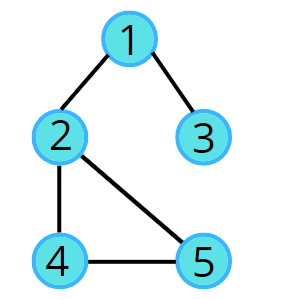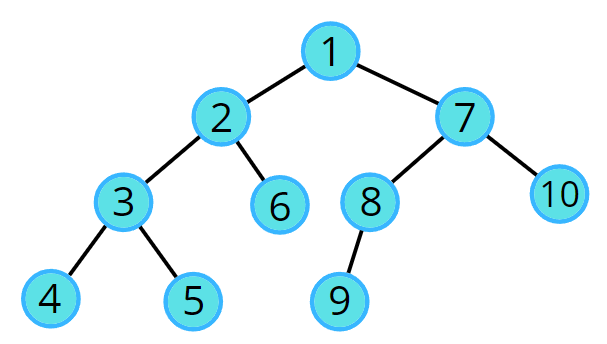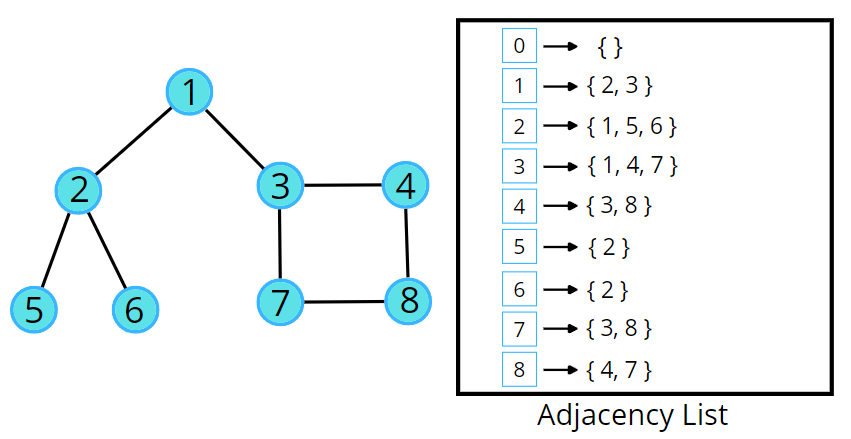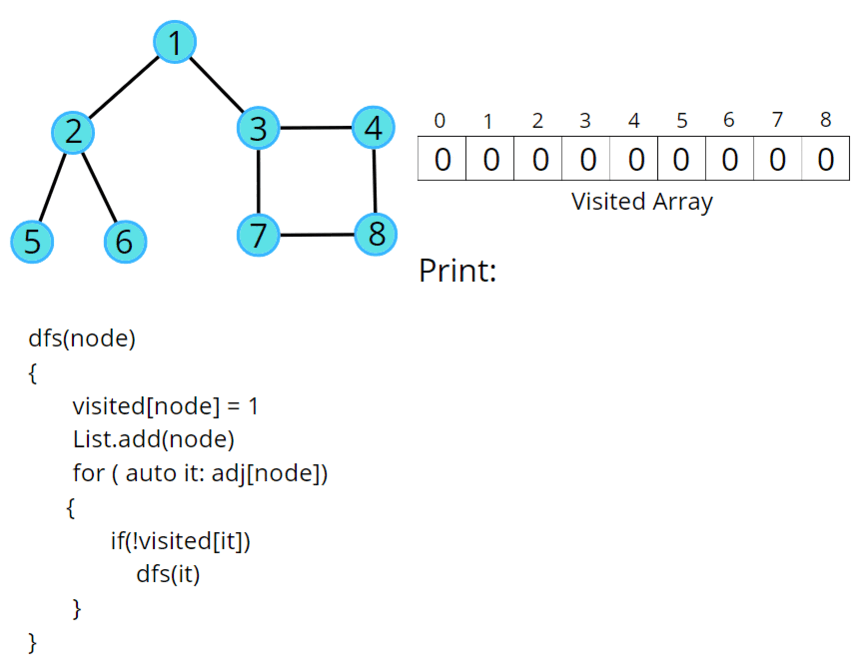# Depth First Search (DFS)

Problem Statement: Given an undirected graph, return a vector of all nodes by traversing the graph using depth-first search (DFS).

Pre-req: Recursion, Graph Representation

Examples:

```Example 1:
Input:Output: 1 2 4 5 3```
```Example 2:
Input:Output: 1 2 3 4 5 6 7 8 9 10```

### Solution

Disclaimer: Don’t jump directly to the solution, try it out yourself first.

### Approach:

DFS is a traversal technique which involves the idea of recursion and backtracking. DFS goes in-depth, i.e., traverses all nodes by going ahead, and when there are no further nodes to traverse in the current path, then it backtracks on the same path and traverses other unvisited nodes.

1. In DFS, we start with a node ‘v’, mark it as visited and store it in the solution vector. It is unexplored as its adjacent nodes are not visited.
2. We run through all the adjacent nodes, and call the recursive dfs function to explore the node ‘v’ which has not been visited previously. This leads to the exploration of another node ‘u’ which is its adjacent node and is not visited.
3. The adjacency list stores the list of neighbours for any node. Pick the neighbour list of node ‘v’ and run a for loop on the list of neighbours (say nodes ‘u’ and ‘w’ are in the list). We go in-depth with each node. When node ‘u’ is explored completely then it backtracks and explores node ‘w’.
4. This traversal terminates when all the nodes are completely explored.

In this way, all the nodes are traversed in a depthwise manner.Note: For a better understanding of the dry run please check the video listed below.

Code:

## C++ Code

``````#include <bits/stdc++.h>
using namespace std;

class Solution {
private:
void dfs(int node, vector<int> adj[], int vis[], vector<int> &ls) {
vis[node] = 1;
ls.push_back(node);
// traverse all its neighbours
for(auto it : adj[node]) {
// if the neighbour is not visited
if(!vis[it]) {
dfs(it, adj, vis, ls);
}
}
}
public:
// Function to return a list containing the DFS traversal of the graph.
vector<int> dfsOfGraph(int V, vector<int> adj[]) {
int vis[V] = {0};
int start = 0;
// create a list to store dfs
vector<int> ls;
// call dfs for starting node
dfs(start, adj, vis, ls);
return ls;
}
};

void addEdge(vector <int> adj[], int u, int v) {
}

void printAns(vector <int> &ans) {
for (int i = 0; i < ans.size(); i++) {
cout << ans[i] << " ";
}
}

int main()
{

Solution obj;
vector <int> ans = obj.dfsOfGraph(5, adj);
printAns(ans);

return 0;
}
``````

Output: 0 2 4 1 3

Time Complexity: For an undirected graph, O(N) + O(2E), For a directed graph, O(N) + O(E), Because for every node we are calling the recursive function once, the time taken is O(N) and 2E is for total degrees as we traverse for all adjacent nodes.

Space Complexity: O(3N) ~ O(N), Space for dfs stack space, visited array and an adjacency list.

## Java Code

``````import java.util.*;
class Solution {

public static void dfs(int node, boolean vis[], ArrayList<ArrayList<Integer>> adj,
ArrayList<Integer> ls) {

//marking current node as visited
vis[node] = true;

//getting neighbour nodes
for(Integer it: adj.get(node)) {
if(vis[it] == false) {
dfs(it, vis, adj, ls);
}
}
}
// Function to return a list containing the DFS traversal of the graph.
public ArrayList<Integer> dfsOfGraph(int V, ArrayList<ArrayList<Integer>> adj) {
//boolean array to keep track of visited vertices
boolean vis[] = new boolean[V+1];
vis = true;
ArrayList<Integer> ls = new ArrayList<>();
dfs(0, vis, adj, ls);
return ls;
}

public static void main(String args[]) {

ArrayList < ArrayList < Integer >> adj = new ArrayList < > ();
for (int i = 0; i < 5; i++) {
}

Solution sl = new Solution();
ArrayList < Integer > ans = sl.dfsOfGraph(5, adj);
int n = ans.size();
for(int i = 0;i<n;i++) {
System.out.print(ans.get(i)+" ");
}
}
}
``````

Output: 0 2 4 1 3

Time Complexity: For an undirected graph, O(N) + O(2E), For a directed graph, O(N) + O(E), Because for every node we are calling the recursive function once, the time taken is O(N) and 2E is for total degrees as we traverse for all adjacent nodes.

Space Complexity: O(3N) ~ O(N), Space for dfs stack space, visited array and an adjacency list.

Special thanks to Vanshika Singh Gour for contributing to this article on takeUforward. If you also wish to share your knowledge with the takeUforward fam, please check out this articleIf you want to suggest any improvement/correction in this article please mail us at [email protected]